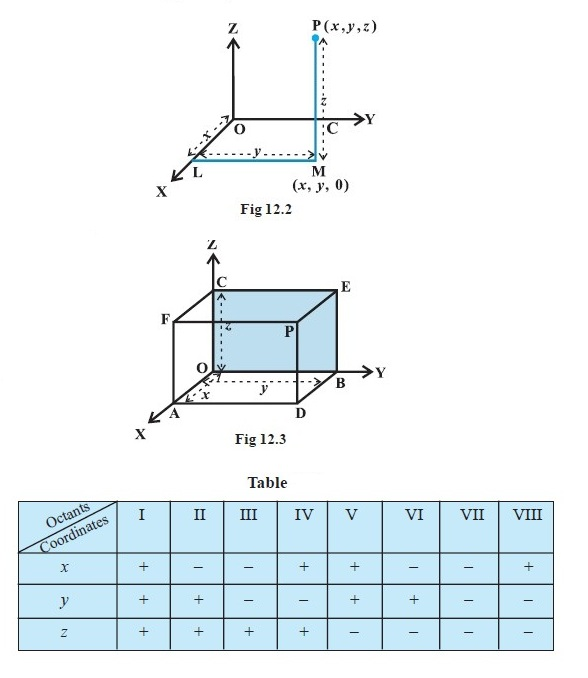Mathematics Introduction and Coordinates of a Point in Space
Click for Only Video

### Topics Coveredstar Introduction
star Coordinate Axes and Coordinate Planes in Three Dimensional Space
star Coordinates of a Point in Space

### Introduction

\color{red} ✍️  You may recall that to locate the position of a point in a plane, we need two intersecting mutually perpendicular lines in the plane. These lines are called the color(blue)"coordinate axes" and the two numbers are called the color(blue)"coordinates of the point with respect to the axes."

\color{red} ✍️  In actual life, we do not have to deal with points lying in a plane only. For example, consider the position of a ball thrown in space at different points of time or the position of an aeroplane as it flies from one place to another at different times during its flight.

### Coordinate Axes and Coordinate Planes in Three Dimensional Space\color{red} ✍️  Consider three planes intersecting at a point O such that these three planes are mutually perpendicular to each other (Fig 12.1).

\color{red} ✍️  These three planes intersect along the lines X′OX, Y′OY and Z′OZ, color(blue)"called the x, y and z-axes", respectively.

\color{red} ✍️  We may note that these lines are mutually perpendicular to each other. These lines constitute the rectangular coordinate system.

\color{red} ✍️  The planes XOY, YOZ and ZOX, called, respectively the XY-plane, YZ-plane and the ZX-plane, are color(blue)"known as the three coordinate planes."

\color{red} ✍️  The distances measured from XY-plane upwards in the direction of OZ are taken as positive and those measured downwards in the direction of OZ′ are taken as negative.

\color{red} ✍️  Similarly, the distance measured to the right of ZX-plane along OY are taken as positive, to the left of ZX-plane and along OY′ as negative,

\color{red} ✍️  In front of the YZ-plane along OX as positive and to the back of it along OX′ as negative.

\color{red} ✍️  The point O is called the color(red)"origin of the coordinate system."

\color{red} ✍️  The three coordinate planes divide the space into eight parts color(blue)("known as octants.")

These octants could be named as XOYZ, X′OYZ, X′OY′Z, XOY′Z, XOYZ′, X′OYZ′, X′OY′Z′ and XOY′Z′. and denoted by I, II, III, ..., VIII , respectively.

### Coordinates of a Point in Space\color{red} ✍️  Given a point P in space, we drop a perpendicular PM on the XY-plane with M as the foot of this perpendicular (Fig 12.2).

\color{red} ✍️  Then, from the point M, we draw a perpendicular ML to the x-axis, meeting it at L.

\color{red} ✍️  Let OL be x, LM be y and MP be z. Then x,y and z are called the x, y and z color(blue)("coordinates"), respectively, the octant XOYZ and so all x, y, z are positive.

\color{red} ✍️  If P was in any other octant, the signs of x, y and z would change accordingly.

\color{red} ✍️  Thus, to each point P in the space there corresponds an ordered triplet (x, y, z) of real numbers.

color(red)("Alternatively"), through the point P in the space, we draw three planes parallel to the coordinate planes, meeting the x-axis, y-axis and z-axis in the points A, B and C, respectively (Fig 12.3).

Let OA = x, OB = y and OC = z. Then, the point P will have the coordinates x, y and z and we write P (x, y, z).

color(red)("Conversely"), given x, y and z, we locate the three points A, B and C on the three coordinate axes.

Through the points A, B and C we draw planes parallel to the YZ-plane, ZX-plane and XY-plane, respectively.

The point of interesection of these three planes, namely, ADPF, BDPE and CEPF is obviously the point P,  corresponding to the ordered triplet (x, y, z).

We observe that if P (x, y, z) is any point in the space, then x, y and z are perpendicular distances from YZ, ZX and XY planes, respectively.

color(red)"Note : " The coordinates of the origin O are (0,0,0).

The coordinates of any point on the x-axis will be as (x,0,0) and the coordinates of any point in the YZ-plane will be as (0, y, z).

color(red)"Remark " The sign of the coordinates of a point determine the octant in which the point lies. The following table shows the signs of the coordinates in eight octants.
Q 3069534415In Fig if P is (2,4,5), find the coordinates of F.Solution:

For the point F, the distance measured along OY is zero. Therefore, the coordinates of F are (2,0,5).
Q 3029634511Find the octant in which the points (–3,1,2) and (–3,1,– 2) lie.Solution:

From the Table the point (–3,1, 2) lies in second octant and the point (–3, 1, – 2) lies in octant VI.